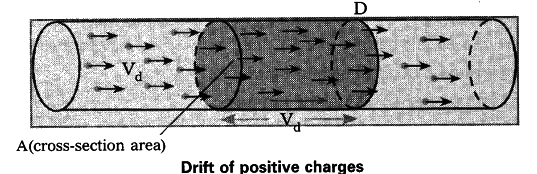# Derive an expression to find drift velocity of electrons

1. Consider a conductor with cross-sectional area A. Assume that the two ends of the conductor are connected to a battery to make the current flow through it.
2. Let ‘{{V}_{d}}’ be the drift speed of the charges and ‘n’ be the number of charges present in the conductor in an unit volume.
3. The distance covered by each charge in one second is ‘{{V}_{d}}’.
4. Then the volume of the conductor for this distance = A{{V}_{d}}
5. The number of charges contained in that volume = n.A{{V}_{d}}
6. Let q be the charge of each carrier.
7. Then the total charges crossing the cross-sectional area at position D in one second is 'nq A{{V}_{d}}'.
This is equal to electric current.
.-. Electric current I = nq A{{V}_{d}}.
Therefore , {{V}_{d}} = 1/nqA